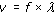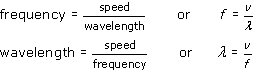### OU short coursesSound for music technology: An introduction

Start this free course now. Just create an account and sign in. Enrol and complete the course for a free statement of participation or digital badge if available.

# 4.2 Frequency, wavelength and the speed of sound

The speed of sound has a joint relationship with both the wavelength and the frequency of the sound. To see why, recall that at the end of Section 2.5, in connection with the wave produced by a tuning fork, I said ‘in the time it has taken for the source to go through one cycle of oscillation, the wave has travelled a distance equal to one wavelength …’.

The time taken for the source to perform one cycle of oscillation is its period T. So, in one period of oscillation, the wave travels a distance λ. To determine the speed of the wave, we need to know how far it travels in a second, rather than in one period. In a second there are f cycles of oscillation, where f is the frequency, so in one second the wave travels f times as far as it travels during just a single cycle of oscillation. Thus:

speed = frequency × wavelength

Or, if we let the speed be represented by v:This can be restated in two other ways:Note that when using these equations in calculations, it is necessary to specify wavelengths in metres and frequencies in hertz (rather than kilohertz or megahertz) in order to be consistent with a speed expressed in metres per second.

## Activity 16 (Self-Assessment)

A tuning fork has a frequency of 384 Hz. Is the wavelength of the sound it produces greater than 1 metre or less than 1 metre? Take the speed of sound to be 340 metres per second. No calculator is needed.